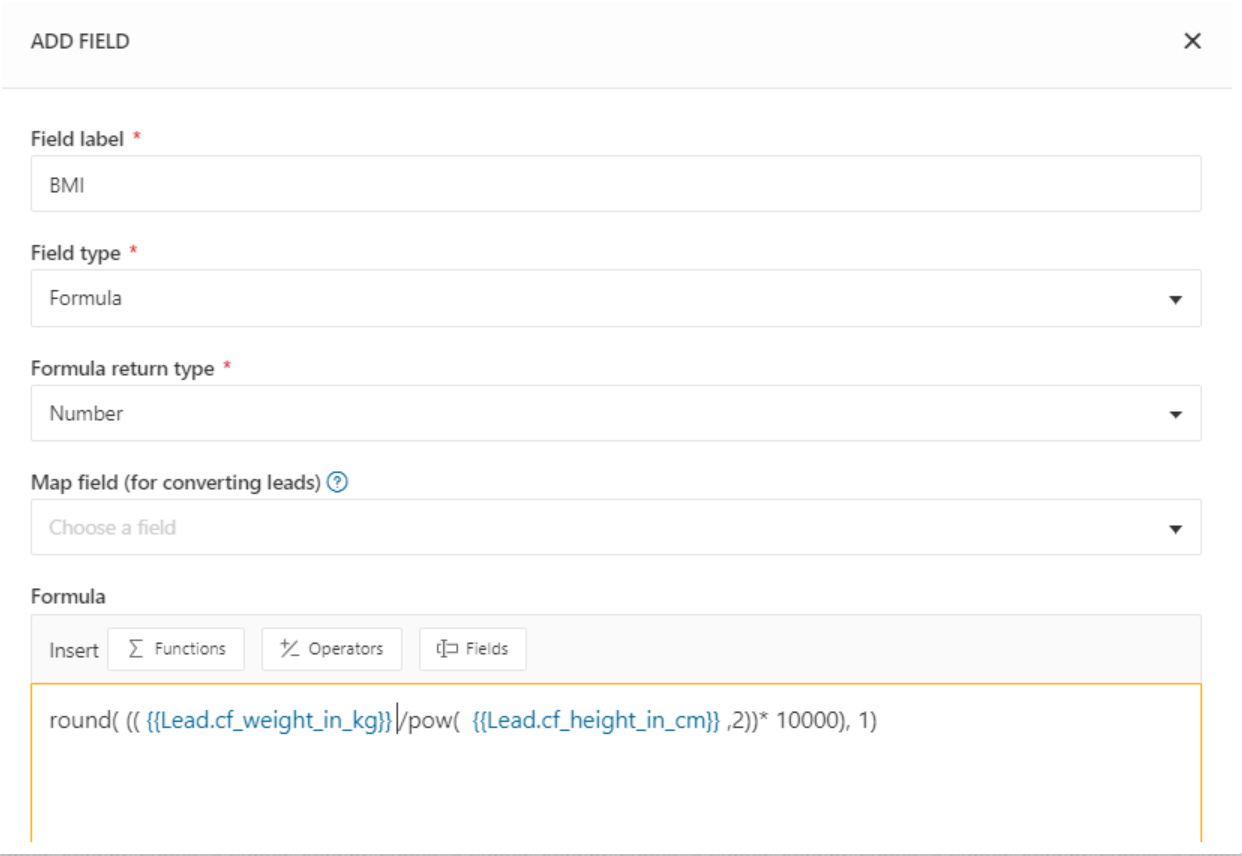With the metric system, the formula for BMI is weight in kilograms divided by height in meters squared. Since we are using height in cm, we would have to divide weight (kg) by height (cm) squared, multiplied by 10,000 and round it to one decimal place.